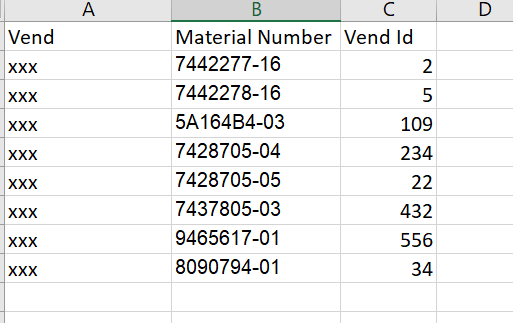# Excel comparison & if matches update in Excel

Hi All,

I am having 2 excel file. In Excel 1 I have (Vend Material Number Vend Id) Columns.
In Excel 2 I have (Vend Id), If vend id in excel 2 matches with excel1 Get the material number from Excel 1 and update in Excel 2.

Excel 1 :Excel 2: (Required Output)There are more than 10k rows, I need to find match & update without using Loop.

Kindly, suggest some solution for this.

Hi @nithya

1. Read the excel1 date using read range and store in a datatable - dt1 (variable name)

2. Read the excel2 date using read range and store in a datatable - dt2 (variable name)

3. Use join datatable activity,

In datatable1 give dt2
In datatable2 give dt1

Give join type as left.

Thanks

But in excel 2 I need to update only Material number column, In Excel 1 I have many columns, Just for reference have mentioned 3 column name.

Hi,

if you have so many rows and you dont want loop concept go with vlookup formula.

use write cell to write the vlookup formula in the excel2 A2 cell to get the Vend Id from the excel 1. After that use Auto fill range to fill the appropriate cells in the column1 of excel 2 with the Vend ID. Hope this logic will help you to achieve your requirement. Thanks.

Hi @nithya

Invoke code arguments

``````For Each dtRow As datarow In dt2.Rows
If dt1.AsEnumerable.Where(Function(row) row("Vend Id").ToString.Trim.Equals(dtRow("Vend Id").ToString)).Count > 0 Then
dtRow("Material Number") = dt1.AsEnumerable.Where(Function(row) row("Vend Id").ToString.Trim.Equals(dtRow("Vend Id").tostring))(0)("Material Number").tostring
End If
Next dtRow
``````

Thanks

@prasath_S Thanks for reply, it worked…

1 Like

This topic was automatically closed 3 days after the last reply. New replies are no longer allowed.